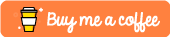#Implementing The Bubble Sort Algorithm in Python

Note - This tutorial was built on top of Python 3.6

In this tutorial we are going to be taking a quick look at the bubble sort sorting algorithm. This algorithm is another very well known sorting algorithm that is quite often referenced in technical interviews. It is amongst the worst performing sorting algorithm with an average and a worst case sorting complexity of `O(N^2)`.

# How It Works

Bubble sort works by constantly iterating through an unsorted array and swapping values within that array until such times as no swaps are made within a full pass through the array.

# Implementing in Python

Now that we have an appreciation for how the algorithm works, let’s now take a look at how you would go about implementing this in the Python programming language.

``````# Bubble Sort In Python
#
# Performance Complexity = O(n^2)
# Space Complexity = O(n)

def bubbleSort(my_list):
# default the swapped variable to true
swapped = True
# kick off our sorting loop
while swapped:
# set swapped to false
swapped = False
# for every element in your list
for i in range(len(my_list)-1):
# if this element is greater than the next
# element
if my_list[i] > my_list[i+1]:
# swap the values
my_list[i], my_list[i+1] = my_list[i+1], my_list[i]
# print out that we've swapepd these values
print("Swapped: {} with {}".format(my_list[i], my_list[i+1]))
# set swapped to true
swapped = True
# finish up by returning our swapped list
return my_list

my_list = [8,2,1,3,5,4]

print(bubbleSort(my_list))``````

# Output

When we go to run this in our terminal, we should see that it successfully swaps a number of elements in our list until there are no more swaps to do and it will return a sorted list.

`````` \$ python3.6 bubbleSort.py
Swapped: 2 with 8
Swapped: 1 with 8
Swapped: 3 with 8
Swapped: 5 with 8
Swapped: 4 with 8
Swapped: 1 with 2
Swapped: 4 with 5
[1, 2, 3, 4, 5, 8]``````

# Conclusion

Awesome! So, in this tutorial, we looked at how you could successfully implement the Bubble Sort algorithm in Python.

Note - If you found this tutorial useful or require further help then please feel free to let me know in the comments section below!